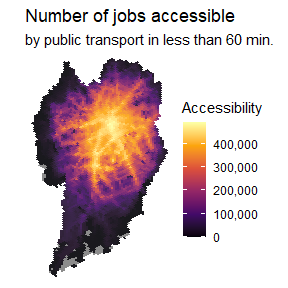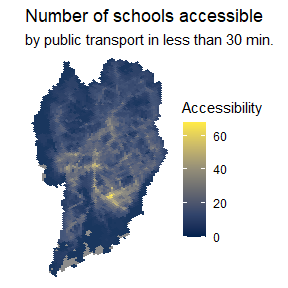# Mapping urban accessibility

#### 2022-08-31

Abstract

Mapping urban accessibility

library(aopdata)
library(sf)
library(ggplot2)
library(data.table)
library(scales)
#> Warning: package 'scales' was built under R version 4.1.3

# download aop data
mode='public_transport',
year=2019,
peak = FALSE,
geometry = TRUE,
showProgress = FALSE)

ggplot() +
geom_sf(data=df, aes(fill=CMATT60), color=NA, alpha=.9) +
scale_fill_viridis_c(option = "inferno", labels = scales::comma) +
labs(title='Number of jobs accessible', fill="Accessibility",
subtitle='by public transport in less than 60 min.') +
theme_void()ggplot() +
theme_void()